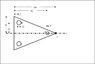# Mass Moment of Inertia of Triangle about its tip

• luznyr
In summary, luznyr tried to find the mass moment of inertia about the tip of a triangle, but wasn't sure how to do it. He found that the letter T is the vertex of the triangle, and used the parallel axis theorem to find the new axis. Excellent work!f

## Homework Statement

Find the mass moment of inertia about the tip T (into the page). All dimensions are in mm, and the density is 7820 kg/m^3. The thickness of the triangle is 10mm

## The Attempt at a Solution

I tried to do a triple integration over dV (as density is constant) and made r^2=x^2+y^2, then multiplied my final answer by the density. I used limits of 0 to 0.01 for dz, 0 to 0.1 for dx and -0.4x to 0.4x for dy. I'm not really sure if this was the correct method, does anyone have any other ideas? Thanks in advance. [my answer was 1.647x10^(-3) kgm^2]

## The Attempt at a Solution

#### Attachments

•question.JPG
16.9 KB · Views: 6,017
I do not see the letter T. Is this the vertex on the line of symmetry with the black dot?

There are standard formulae for mass moments of inertia (MMI) of triangles and circles about their centroids. You can apply the parallel axis theorem to transform the MMI into the new axis, unless it is coursework on calculus, in which case you may need to go through all the motions of integration.

Google mass moments of intertia and see if it gets you anywhere.

Last edited:
Yes, the letter T is the vertex of the triangle. It's not for a calculus course but the problem states that we need to use integration.

alright thanks for that. the removal of the holes was a later part of the question, and the parallel axis theorem can be used for that.

Hi luznyr,

Nice work :) I'm just a bit confused here, because I did it by another method and got a slightly different answer..

Consider a thin vertical strip with area dA = x / 100 * 80 * dx
therefore volume dV = x / 100 * 80 * dx * t

and IT = integral ( r^2 dm) over volume
= integral (rho * r^2 * dV) over volume
= integral (x / 100 * 80 * t * x^2 * dx), x = 0 to 100
= 0.001572 kgm^2

I would appreciate a clarification ;)

alias99: Although the problem statement in post 1 is unclear, luznyr is asking for the polar mass moment of inertia about a z axis passing through point T. The z axis is normal to the plane of the page. Your derivation is correct for the rectangular mass moment of inertia about a y-axis passing through point T (except you inadvertently omitted rho from one of your equations, and secondly, your answer is slightly inaccurate and should instead be 0.001564 kg*m^2.)

can u show exactly how u did it? I am curious haha.. triple integration about dV..

nvn, you're right.. I made 3 mistakes1. should be Z-axis through T
2. I missed rho in an equation..haha..
3. I used 7860 instead of the correct density, 7820

I did it by luznyr's method and got his answer..Thank you very much for your help nvn, you saved me